# Electrical Engineering test questions for exams and entrance - 5

## Electrical Engineering test questions for exams and entrance - 5

(1) For a speed control application, an ac induction motor is driven from an inverter with a constant V/f control. The inverter output frequency is 40Hz and with half the rated slip. The

details of the motor name-plate details are as follows:

V: 415 VPh: 3 f: 50Hz N: 2850rpm

The running speed of the motor is

(A) 2000rpm
(B) 2560 rpm
(C) 2400 rpm
(D) 2340 rpm

(2) Which transmission line distance protection relay has the property of being inherently directional?

(A) MHO relay
(B) OHM relay
(C) Reactance relay
(D) Impedance relay

(3) Consider the transmission line of 400Kv and the surge impedance of 250Ω. A lightning stroke discharges impulse current of 10kA (peak) on the transmission line. What should be the

magnitude of transient over-voltage travelling waves in either direction assuming equal distribution from the point of lightning strike?

(A) 1200 Kv
(B) 1250 Kv
(C) 2000 Kv
(D) 2500Kv

(4) Figure shows the torque speed characteristic of an induction motor. W, X, Y, Z represents four points of operation. The point of operation where slip greater than 1 is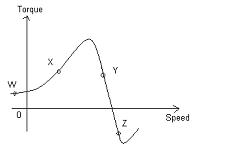(A) W
(B) X
(C) Y
(D) Z

(5) The figure shown is used to measure resistance R. The Resistance of ammeter is 0.01 Ω and that of voltmeter is 2000 Ω. The reading of ammeter is 2 A and that of voltmeter is

180 V giving a measured resistance of 90 Ω. The percentage error in the measurement is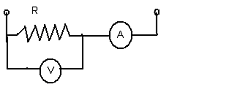(A) 3.5%
(B) 4.5%
(C) 2.5%
(D) 1.5%

For Questions 6 and 7 refer to the data given below:

A 300KVA transformer has 95% efficiency at full load, 0.8 pf lagging and 96% efficiency at half load, unity pf.

(6) Under full load operation iron loss in KW will be

(A) 9.12
(B) 2.31
(C) 3.45
(D) 4.12

(7) Maximum efficiency (in %) at unity pf load is

(A) 95.2
(B) 97.2
(C) 98.2
(D) 96.2

(8) The figure shows the block diagram of a closed loop control system. For the system to have damping ratio of 0.7 and an undamped natural frequency of 5 rad/sec, what should be the

respective values of K and P?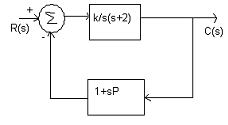(A) K = 15 and P = 0.2
(B) K = 15 and P = 0.3
(C) K = 25 and P = 0.2
(D) K = 25 and P = 0.5

ANSWER: K = 25 and P = 0.2

(9) If a synchronous generator is feeding a zero power factor (lagging) load at rated current then the armature reaction is

(A) Ineffective
(B) Cross-Magnetizing
(C) Magnetizing
(D) Demagnetizing

(10) Consider an electric motor that starts with a load torque of 7Nm on its shaft. It develops a starting torque of 15Nm. The systems moment of inertia if the acceleration at start is 2

rad/sec² (neglecting viscous and coulomb friction) must be

(A) 4 Kgm²
(B) 5 Nm²
(C) 0.25 Kgm²
(D) 1 Nm²

(11) For the function f (x) = sin²x, the Fourier series is

(A) 0.5 – 0.5 cos 2x
(B) sin x + sin 2x
(C) cos x + cos 2x
(D) sin 2x + cos 2x

ANSWER: 0.5 – 0.5 cos 2x

(13) Consider a moving coil of the meter be positioned in a uniform radial flux density of 200 mT and carries a current of 50 mA. It has 100 turns, 10mm in length and 20 mm in depth. The

torque on the coil is

(A) 100 μNm
(B) 200 μNm
(C) 2 μNm
(D) 1 μNm

(14) The program written below is a software delay subroutine.

DELAY: MVI H, 255D
MVI L, 255D
LOOP: DCR L
JNZ LOOP
DCR H
JNZ LOOP
DCR L instruction will be executed

(A) 255 times
(B) 510 times
(C) 65279 times
(D) 65025 times

(15)

Let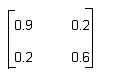be the Z matrix of a 2-port network. For the same network the Y22 element of the corresponding Y matrix will be

(A) -0.6
(B) 0.8
(C) 1.8
(D) 1.4

(16) Let a 230V, 50 Hz, 4-pole single-phase induction motor is rotating in the clockwise (forward direction) at a speed of 1425 rpm. What will be the effective rotor resistance in the

backward branch of the equivalent circuit if the rotor resistance at standstill is 7.8Ω?

(A) 1 Ω
(B) 4 Ω
(C) 50 Ω
(D) 200 Ω

(17) Consider a surface S (x, y) = 2x +5y – 3. This surface is integrated once over a path that consist of the points and the points satisfy (x + 1) 2 + (y –1) 2 = √
. The integral evaluates to

(A) 0
(B) 1
(C) Infinite
(D) None of the above

(18) Which one of the following holds true for a single-phase capacitor start induction motor?

(A) Interchange the supply terminals to change the direction of rotation
(B) Reverse the main winding terminals to change the direction of rotation.
(C) The direction of rotation cannot be changed.
(D) To improve power factor capacitor is used.

ANSWER: Reverse the main winding terminals to change the direction of rotation.

(19) X is a real number of the function f(x) = (x² -4) ². This function has

(A) Only one maxima
(B) Only three minima
(C) Only one minima
(D) Only two minima

(20) Let G(s) = as + 1 / s² be the open loop transfer function of a unity feedback control system. To give phase margin of 45°, the value of a is

(A) 0.841
(B) 0.123
(C) 0.736
(D) 1.211

(21) Consider a system whose transfer function is 100/s² + 20s +100. This system is

(A) An unstable system
(B) An overdamped system
(C) A critically damped system
(D) An underdamped system

(22) The rated voltage of a 500 Mw 3-phase Y-connected synchronous generator is of 21.5Kv at 0.85pf. What will be the line current when operating at full load rated conditions?

(A) 23.16 KA
(B) 25.19 KA
(C) 13.57 KA
(D) 15.79 KA

(23) For the HEX number AB.CD, the octal equivalent is

(A) 526.582
(B) 526.439
(C) 253.632
(D) 253.314

For questions 24 and 25 refer to the data given below: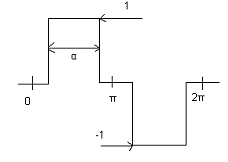Figure shows the periodic output voltage of an inverter.

(24) The rms fundamental component of the output voltage, when the conduction angle a = 120° is

(A) 1.55 V
(B) 0.78 V
(C) 1.12 V
(D) 0.56 V

(25) What should be the value of a for the output of the converter to be free from 5th harmonic?

(A) 90°
(B) 72°
(C) 36°
(D) 150°